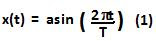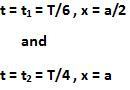## Saturday, May 29, 2010

### Irodov Problem 4.5

The equation for simple harmonic oscillations of amplitude a and time period T is given by,The mean velocity over a time interval is the displacement over the time interval over the total time elapsed during the time interval given by,From (1) we can see that the oscillating particle will reach half the amplitude (by setting x=a/2) within t1 = T/6 seconds and it will reach the extreme position (setting x = a) in t2=T/4 seconds. This is also shown in the figure. Hence, we have,Thus we can compute the mean velocities in question as,1.you can say i'm as dumb as fuck, but no way i can understand how you got t1=T/6
a/2=a*sin(2*pi*t/T)
1/2=sin(2*pi*t/T)
1/2=sin(pi/6)
2*pi*t/T=pi/6
t=(T*pi)/(2*pi*6)
t=T/12
what am i doing wrong? >_<
(obviously 6a/T is the right answer, but... )

2.Be care ful, in the book of Irodov use tehe equetion: x = acos(xt + c). in this web Krishna Kant Use X = aSen(xt + c). both asume tha c= 0, because it is not importan, but, you can not discribe the same problem con diferente equetion.

for the case that you use X = asen(xt), the t1 = T/12, but if you use X = aCos(xt), the t1 = T/6 and you will find de correct answer.

the mind problem, is that is not given the constan *c*.

Krishna Kant review that.

I apreciate you helps to

3.4.5.